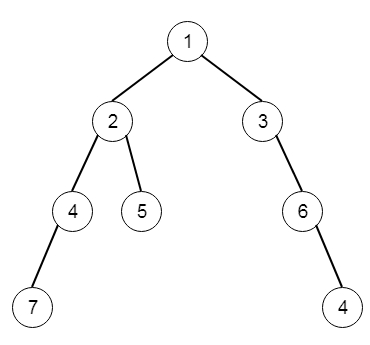# Program to find sum of the deepest nodes in C++

Suppose we have a binary tree; we have to find the sum of values of its deepest leaves. So if the tree is like −Then the output will be 11.

To solve this, we will follow these steps −

• Define a map m, and maxDepth

• Define a recursive method solve(), this will take node and level, initially level is 0

• if node is not present, then return

• maxDepth := max of level and maxDepth

• increase m[level] by value of node

• solve(left of node, level + 1)

• solve(right of node, level + 1)

• In the main method, setup maxDepth := 0, then solve(root, 0)

• return m[maxDepth]

Let us see the following implementation to get better understanding −

## Example

Live Demo

#include <bits/stdc++.h>
using namespace std;
class TreeNode{
public:
int val;
TreeNode *left, *right;
TreeNode(int data){
val = data;
left = NULL;
right = NULL;
}
};
class Solution {
public:
int maxDepth;
map <int, int> m;
void solve(TreeNode* node, int level = 0){
if(!node)return;
maxDepth = max(level, maxDepth);
m[level] += node->val;
solve(node->left, level + 1);
solve(node->right, level + 1);
}
int deepestLeavesSum(TreeNode* root) {
maxDepth = 0;
m.clear();
solve(root);
return m[maxDepth];
}
};
main(){
TreeNode *root = new TreeNode(1);
root−>left = new TreeNode(2);
root−>right = new TreeNode(3);
root−>left−>left = new TreeNode(4);
root−>left−>right = new TreeNode(5);
root−>right−>right = new TreeNode(6);
root−>right−>right−>right = new TreeNode(4);
root−>left−>left−>left = new TreeNode(7);
Solution ob;
cout << (ob.deepestLeavesSum(root));
}

## Input

TreeNode *root = new TreeNode(1);
root−>left = new TreeNode(2);
root−>right = new TreeNode(3);
root−>left−>left = new TreeNode(4);
root−>left−>right = new TreeNode(5);
root−>right−>right = new TreeNode(6);
root−>right−>right−>right = new TreeNode(4);
root−>left−>left−>left = new TreeNode(7);

## Output

11

Updated on: 21-Oct-2020

61 Views